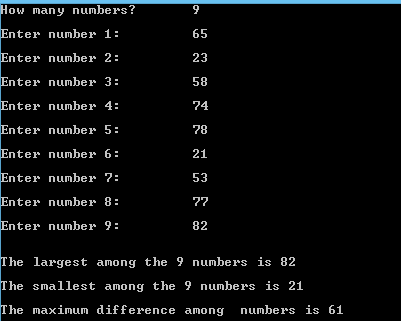## Friday, 9 January 2015

### C Program to find the Maximum difference between all the elements in an array

This is a C Program which finds out the maximum difference between the elements in an array.

Here first we find the largest element in the array and store the result and then find the smallest element in the array and store the result .
Now substract the largest element with the smallest element , this gives us the maximum difference in an array.

PROGRAM :
```#include <stdio.h>
#include <stdlib.h>

int main()
{
int i,n,array,dup,l,s;
printf("How many numbers? \t");
scanf("%d",&n);
for(i=0;i<n;i++)
{
printf("\nEnter number %d: \t",i+1);
scanf("%d",&array[i]);
dup[i]=array[i];    //storing the copy of of array[]
}

for(i=0;i<n;i++) //checking for largest
{
if(array<array[i])
array=array[i];
}
l=array; //storing largest num
printf("\n\nThe largest among the %d numbers is %d\n",n,array);

for(i=0;i<n;i++)  //checking for smallest
{
if(dup>dup[i])  //we used duplicate because original array[] has changed
dup=dup[i];
}
s=dup; //storing smallest
printf("\nThe smallest among the %d numbers is %d\n",n,dup);
printf("\nThe maximum difference among  numbers is %d\n",l-s);
return 0;
}
```

OUTPUT :C - Maximum difference between the elements in an array